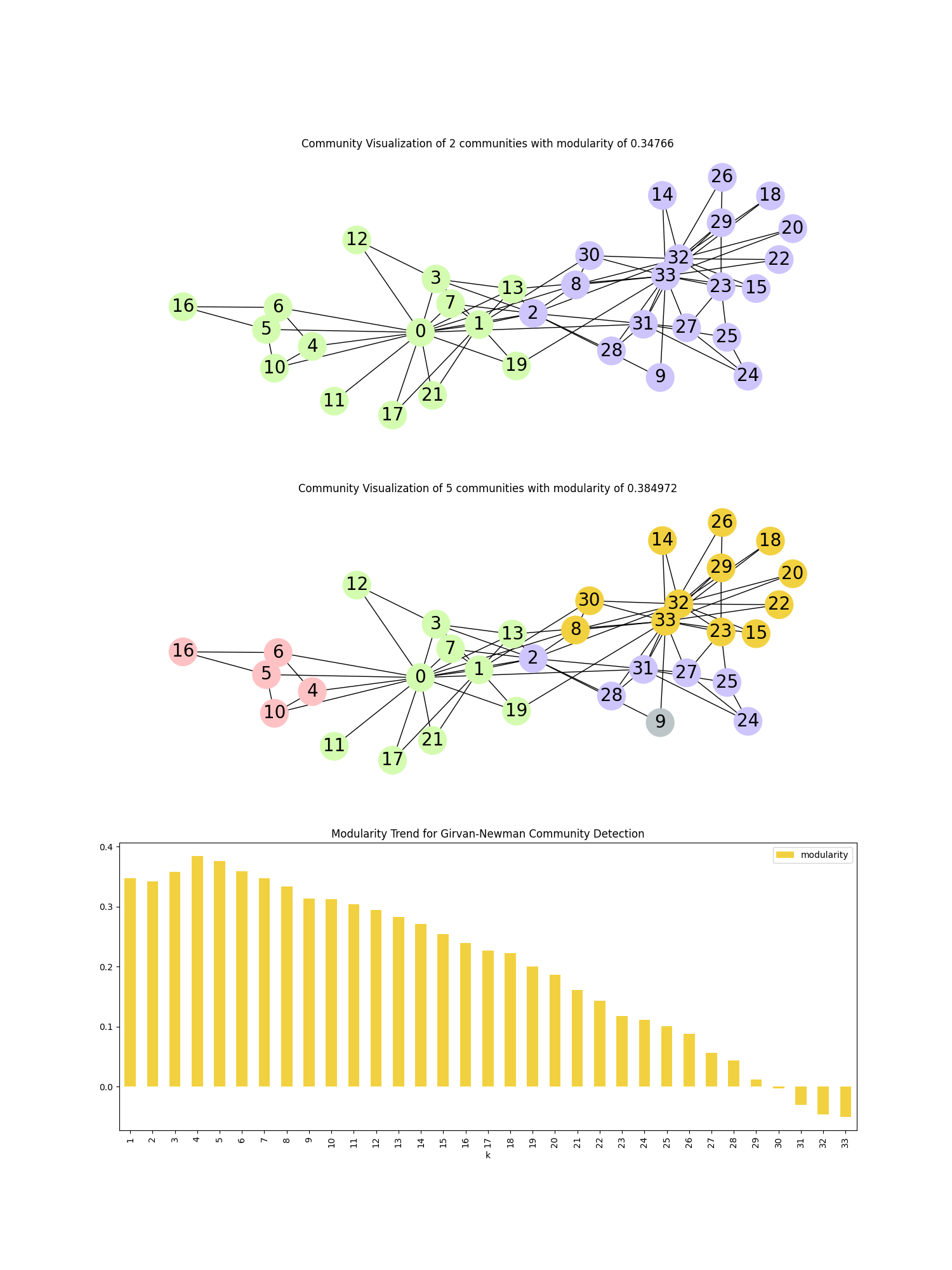# Community Detection using Girvan-Newman#

This example shows the detection of communities in the Zachary Karate Club dataset using the Girvan-Newman method.

We plot the change in modularity as important edges are removed. Graph is coloured and plotted based on community detection when number of iterations are 1 and 4 respectively.```import networkx as nx
import pandas as pd
import matplotlib.pyplot as plt

# Load karate graph and find communities using Girvan-Newman
G = nx.karate_club_graph()
communities = list(nx.community.girvan_newman(G))

# Modularity -> measures the strength of division of a network into modules
modularity_df = pd.DataFrame(
[
[k + 1, nx.community.modularity(G, communities[k])]
for k in range(len(communities))
],
columns=["k", "modularity"],
)

# function to create node colour list
def create_community_node_colors(graph, communities):
number_of_colors = len(communities)
colors = ["#D4FCB1", "#CDC5FC", "#FFC2C4", "#F2D140", "#BCC6C8"][:number_of_colors]
node_colors = []
for node in graph:
current_community_index = 0
for community in communities:
if node in community:
node_colors.append(colors[current_community_index])
break
current_community_index += 1
return node_colors

# function to plot graph with node colouring based on communities
def visualize_communities(graph, communities, i):
node_colors = create_community_node_colors(graph, communities)
modularity = round(nx.community.modularity(graph, communities), 6)
title = f"Community Visualization of {len(communities)} communities with modularity of {modularity}"
pos = nx.spring_layout(graph, k=0.3, iterations=50, seed=2)
plt.subplot(3, 1, i)
plt.title(title)
nx.draw(
graph,
pos=pos,
node_size=1000,
node_color=node_colors,
with_labels=True,
font_size=20,
font_color="black",
)

fig, ax = plt.subplots(3, figsize=(15, 20))

# Plot graph with colouring based on communities
visualize_communities(G, communities, 1)
visualize_communities(G, communities, 2)

# Plot change in modularity as the important edges are removed
modularity_df.plot.bar(
x="k",
ax=ax,
color="#F2D140",
title="Modularity Trend for Girvan-Newman Community Detection",
)
plt.show()
```

Total running time of the script: ( 0 minutes 0.573 seconds)

Gallery generated by Sphinx-Gallery